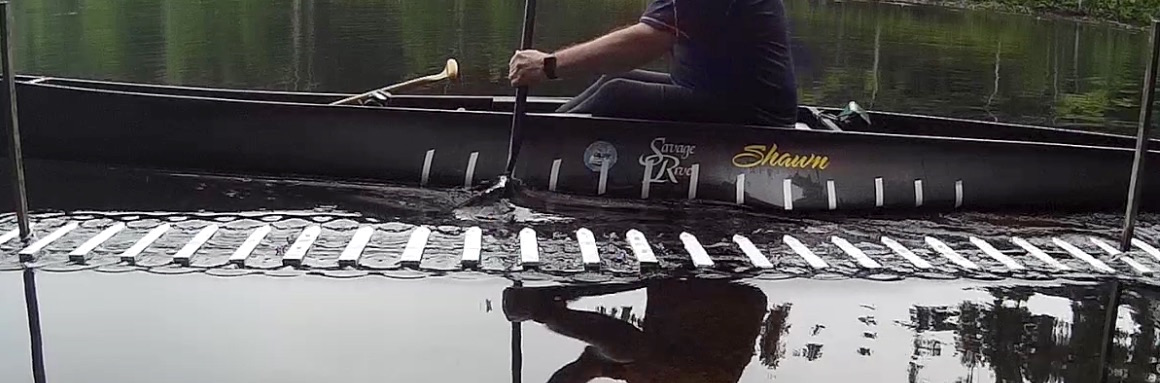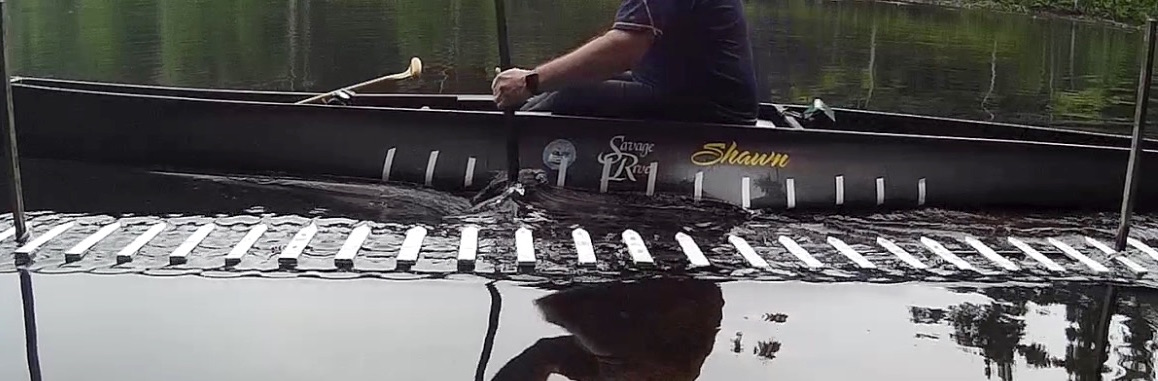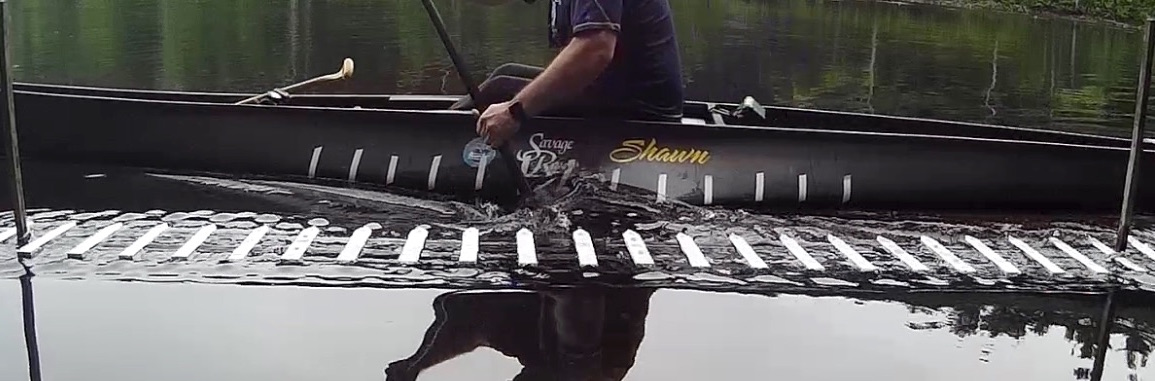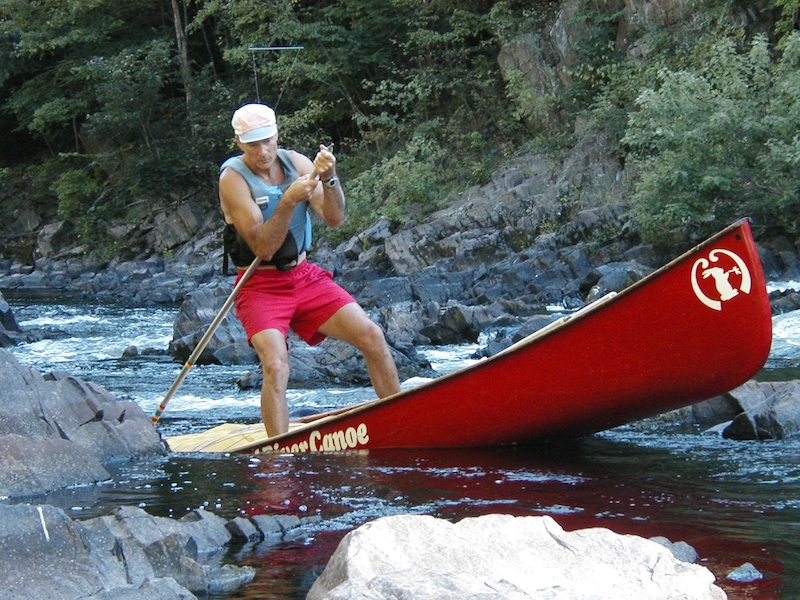# Part 5: What Moves You

by Shawn Burke, Ph.D.

When you paddle, each stroke propels your hull forward against our familiar nemesis, drag. But why does the canoe move forward, and not in some other direction? And more fundamentally, why does it move at all? The concept of momentum provides the answer. And it all can be understood by considering … space boots.

When I was in 6th grade I bought a collection of science fiction stories at a garage sale. One of the tales centered on an astronaut marooned outside his spacecraft; how he became marooned, I don’t recall. Fortunately, he was wearing a space suit – it’s pretty cold in outer space. Unfortunately, the space suit had no thrusters for propelling him back to his craft. And he had a limited supply of air left to breath. How did he get back inside his rocket ship before running out of air? By using science!

Our stranded astronaut recalled that when you fire a rifle, there is recoil. The rifle “throws” a bullet in one direction, and in response the rifle moves in the opposite direction. The astronaut realized that if he threw something in the direction opposite his spacecraft, his body would “recoil” in response and move toward the spacecraft. Knowing that he couldn’t throw his oxygen tanks (gotta breathe), his space helmet (thus avoiding the worst case of brain freeze ever), or his space gloves (he needed functioning hands to open the hatch back into his spacecraft), he took off his space boots and threw them away from his rocket ship as hard as he could. He had a pretty good aim because the rest of him drifted slowly toward the space ship’s hatch, whereupon he was able to climb back inside. All because he remembered physics, and the concept of conservation of momentum. And, of course, because he had space boots.

We’ve all heard the word “momentum,” but what does it means in scientific terms, and how does it apply to paddling? Momentum can be thought of as “mass in motion.” A canoe and its paddler(s) have mass, so if a canoe is moving then it has momentum. An object’s momentum depends upon its mass and its velocity. Formally, momentum – typically written in shorthand as “P” – is equal to the mass of the object times its velocity:$Momentum\equiv P=mass\times velocity$

This equation shows that momentum is linearly proportional to an object’s mass, as well as linearly proportional to its velocity. And by “linearly” we mean that neither quantity appears as a square, cube, square root, etc., but only as itself. This means that if you throw a baseball at 45mph, and a major league pitcher throws the same baseball at 90mph (e.g., twice as fast), then the baseball thrown by the major leaguer has twice as much momentum as when you threw it.

So now consider a canoe paddled by a solo paddler. At the end of each stroke their hull has a certain amount of momentum Phull, which is quantified as the total mass of the hull mhull – including the mass of paddler – times the hull’s velocity vhull:${{P}_{hull}}={{m}_{hull}}\times {{v}_{hull}}$

With each stroke, the paddle blade is accelerating a volume of water. It may seem that your paddle is planted in concrete at the catch, but in truth there is slippage; if you watch the strokes of adjacent paddlers you’ll see little pools of swirling, aft-moving water behind their blades at the conclusion of their power phase. This slip is illustrated in the sequence shown in Figs. 1-5 showing a side view of the power phase. The paddle rotates at and after the catch, and slips sternward in the inertial (Earth-based) reference frame as the water entrained by the blade accelerates.Figs. 1-5: Power phase, side view, with both hull and fixed fiducials.

Your paddle stroke has imparted momentum Pwater to this mass of water mwater, which can be idealized as a plug of water having a velocity vwater, in the direction opposite your hull’s motion. The momentum of this idealized plug of water is then${{P}_{water}}=-{{m}_{water}}\times {{v}_{water}}$

The minus sign indicates that the water’s momentum is in the opposite direction of the hull’s momentum. This is because in physics velocity is a vector quantity, which means velocity has both a magnitude (referred to as “speed”) and a direction as well. The minus sign here indicates that the momentum of the slug of water is opposite to the momentum of the hull since the water and the canoe move in opposite directions.

Physics teaches us that the momentum of a system is conserved. Here, the system is the canoe and paddler, as well as the slug of water “thrown” by the paddle. Consequently, at the conclusion of each stroke the total momentum of the system – here, the canoe/paddler(s) and the water – must equal zero. This may be written as,${{P}_{hull}}+{{P}_{water}}={{m}_{hull}}\times {{v}_{hull}}-{{m}_{water}}\times {{v}_{water}}=0$

As a result, the momentum of the canoe plus paddler, plus the momentum of the slug of water, must be equal. This means$\Rightarrow {{m}_{hull}}\times {{v}_{hull}}={{m}_{water}}\times {{v}_{water}}$

So to move the hull, you impart momentum to the plug of water entrained by your paddle. And the hull will travel in the direction opposite the plug of water that you have “thrown.” This should be familiar to anyone who has paddled a canoe; the hull doesn’t move in the same direction as your paddle blade moves in the water, nor does it move perpendicular to the path of the paddle. And perhaps the most interesting take away, particularly relevant to our discussion, is that the momentum of the water equals the momentum of you and your canoe at the end of each stroke. It’s exactly like our astronaut floating freely in space – to move toward his spacecraft, the astronaut threw his space boots in the direction opposite his ship, and he zoomed off. If he had heavier space boots, or threw them faster, he would move faster in response.

So what does this tell us about moving the canoe faster? Equation (4) shows us how. One can express equation (4) in terms of hull speed vhull as${{v}_{hull}}=\frac{{{m}_{water}}\times {{v}_{water}}}{{{m}_{hull}}}$

To increase hull speed, we see from this equation that there are several options:

• For a fixed paddle size and power phase stroke finishing speed, the numerator in equation is a constant. So to go faster our paddler should get a lighter hull, lose weight, or both since the only remaining variable in this case is the combined mass of the canoe and paddler.
• For a fixed power phase stroke finishing speed, our paddler could move a bigger slug of water by using a paddle with a bigger blade in order to entrain more water with the paddle stroke.
• For a fixed paddle size, our paddler could move the slug of water faster with a faster stroke power phase finishing speed, or use a smaller paddle but have an even faster power phase finishing speed.
• Or, our paddler could lose weight, buy a lighter hull, get a paddle with a bigger blade, and move that blade faster. Phew! I’m tired already, not to mention broke from the cost of all the new gear.

Practically speaking, each option will appeal to specific paddlers based upon energy, paddle force, and power considerations. We’ll address energy issues below; paddle force and power will be addressed in a subsequent Science of Paddling article.

Now what if the canoeist isn’t paddling, but instead is poling? As you may (or may not) know, canoe polers “stand tall in their canoes” and propel themselves using a pole of wood or aluminum, pushing the pole into the riverbed and pushing off as illustrated by 12-time ACA poling champion Harry Rock:Fig. 6: Poling up a drop.

Assuming the pole is well planted and doesn’t slip, the poler is pushing against the mass of the Earth. In this case our equation for momentum conservation takes a particularly interesting form:${{P}_{hull}}+{{P}_{Earth}}={{m}_{hull}}\times {{v}_{hull}}-{{m}_{Earth}}{{v}_{Earth}}=0$

So each time the poler plants their pole and propels their canoe forward, they are changing the momentum of the Earth, pushing it in the opposite direction!

As noted above, a canoe can attain a particular speed by using the paddle to move a larger mass of water slowly, or a smaller mass of water more quickly. The choice is yours as long as total momentum is conserved, per Equation (4). The difference between these two scenarios becomes more apparent when we consider the energy expended in each scenario, e.g. the cost in energy to you, the paddler.

At the end of each stroke, the energy in the canoe/paddler/water system is due in the motion of the canoe/paddler(s) and the motion of the water. This “motion energy” is called kinetic energy. Kinetic energy is linearly proportional to mass, and proportional to the square of velocity. The total kinetic energy of the system is then expressed as the sum of the kinetic energy of the hull and paddler, and the kinetic energy of the water:${{E}_{kinetic}}=\frac{1}{2}{{m}_{hull}}v_{hull}^{2}+\frac{1}{2}{{m}_{water}}v_{water}^{2}$

Let’s consider the impact of paddle blade size for a given hull speed vhull. We’ll consider two cases: a “smaller” paddle blade with an area represented by A1, and a “bigger” paddle blade with an area represented by A2. We assume that with each stroke the paddle pushes a plug of water having a volume coving the area of the blades, extending a distance T behind the power face – this is merely a simplifying assumption for the purposes of comparing the impact of blade size. This means that at the end of the stroke the paddler moves a mass of water equal to the volume of water (the blade area A time the thickness T) time the density of water$\rho$:${{m}_{water}}=A\times T\times \rho$

For the “smaller blade” paddle, the total system kinetic energy at the end of the stroke is then${{E}_{1}}=\frac{1}{2}{{m}_{hull}}v_{hull}^{2}+\frac{1}{2}\rho {{A}_{1}}Tv_{water,1}^{2}$${{E}_{2}}=\frac{1}{2}{{m}_{hull}}v_{hull}^{2}+\frac{1}{2}\rho {{A}_{2}}Tv_{water,2}^{2}$

The ratio of these two energies, e.g. the ratio of how much energy is expended by using a smaller paddle vs. using a larger paddle to attain the same hull speed, is$\frac{{{E}_{1}}}{{{E}_{2}}}=\frac{\frac{1}{2}{{m}_{hull}}v_{hull}^{2}+\frac{1}{2}\rho {{A}_{1}}Tv_{water,1}^{2}}{\frac{1}{2}{{m}_{hull}}v_{hull}^{2}+\frac{1}{2}\rho {{A}_{2}}Tv_{water,2}^{2}}$

Now it turns out that the second term in both the numerator and the denominator in this equation is larger than the first term. As a result, the ratio can be approximated as$\frac{{{E}_{1}}}{{{E}_{2}}}\approx \frac{{{A}_{1}}v_{water,1}^{2}}{{{A}_{2}}v_{water,2}^{2}}$

One next uses the momentum conservation equation for each scenario to solve for vwater,1 and vwater,2 in terms of the blade areas. After a little bit of algebra, the approximation for the ratio of energies above drastically simplifies to become$\frac{{{E}_{1}}}{{{E}_{2}}}\approx \frac{{{A}_{2}}}{{{A}_{1}}}$